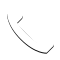Select Page

Do you have a loan and you are wondering if you should repay the loan as soon as possible so that you would not be paying more interest over the course of the loan? Should you keep the current loan and continue to pay a monthly/yearly payment or clear the loan once and for all. Of course, in terms of a real estate mortgage loan, it is often hard for clients to repay the full loan due to the huge capital outlay. Should they pay part of the loan first? This analysis is applicable to all kinds of loan. The key to deciding is by looking at your current loan interest rate and comparing it with the investment return that you are able to get from other investments.

## Current Loan Interest Rate

This is the rate of interest that you will have to pay monthly for your remaining loan balance. You should take note that as you drag your loan term the total interest quantum would increase. For example, if you have a choice to take a loan of \$500,000 for 20 years or 30 years. The total accumulated interest would be more for the 30 years and lesser for the 20 years. However, the monthly payment is lesser for the 30 years loan than the 20 years loan.

## Investment Return

Investment return refers to yield you may get from other investment vehicles such as stocks, bonds, REITS, etc. Different individuals have a different investment yield as such it is subjective. Another way to think is how much rate of return (%) can you get if you invest your current pile of money. This rate of return should be consistent and a simple way would be to average out over 3 years. The rate of return should also be an annual rate of return for easy comparison with the mortgage interest rate.

## Determining Factor

If you are able to get a better rate of return with another investment vehicle, why not invest in that vehicle with your current available cash? Imagine paying back your mortgage loan of \$400,000 with 20years remaining loan term with interest of 2%, this means that you save 2% for the remaining term of the loan. However, if you have another investment vehicle which can provide you with 8% return, the money that was not placed in this investment vehicle means that you are losing out 8% rate of return. With the 2% earned, you end up with a loss of 6% rate of return.

#### Let’s do a calculation for the above scenario to see how much monetary loss is there.

If you continue the loan. For the \$400,000 the monthly payment is (n=240, i/y=2%, p/y=12, PV=\$400,000, FV=0) PMT=-2023.53. Finding out the interest payable, we take the monthly payment and multiply by 240 to get the total payment over 20 years subtracting the 400,000 that we loan. (-2023.53 x 240) + 400,000 = -\$85,648, this is the interest payable over the 20 years. You will only lose \$85,648 as interest to the loan.

If you repay the loan. This means that you will lose 8% rate of return for 20 years for the \$400,000 that you use to repay the loan. Calculating the total interest earning. (n=240, i/y=8%, p/y=12, PV=\$400,000, PMT=0) FV=\$1,970,721.11. The amount from your investment earned over the period of 20 years is \$1,970,721.11 – \$400,000 = \$1,570,721.11. You will lose \$1,570,721.11 if you repay the loan as you will not be able to invest the \$400,000.

### Conclusion

Depending on what you are going to do with the money used the repay the loan, you will be able to decide if you should repay the loan or continue with the loan. If you are going to spend the money or keep it in the bank and not make money work for you, it is better for you to repay the loan to save on the interest. If you have other investment vehicle which is able to provide you with a better rate of return than the loan interest rate, it is better for you to continue with the loan.

## Get In Touch

7 + 8 =Click To Call